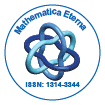# Mathematica EternaOpen Access

ISSN: 1314-3344

## Abstract

### Reverse engineering Turing Machines and insights into the Collatz conjecture.

John Nixon

In this paper I have extended my earlier work  on small Turing Machines (TMs) by developing a method for obtaining recursive definitions of the irreducible regular rules (IRR) for a TM when explicit formulae for them cannot be obtained. This has been illustrated by two examples. The first example was randomly chosen and the second example was designed to simulate the Collatz conjecture. Analysis of this TM based on the its IRR suggested new approaches that might be the basis for a proof of this conjecture. The method involves running the TM backwards from a configuration set (CS). This in general produces a tree of CSs at each step. The aim is to find CS’s y that are reachable from a CS x that simply specifies the symbol about to be read and the machine state. This means that following the computation forward from x by adding some symbols when needed at the pointer, the CS y can be reached. These CS’s form the basis of the LHS’s of the IRR.

Top